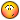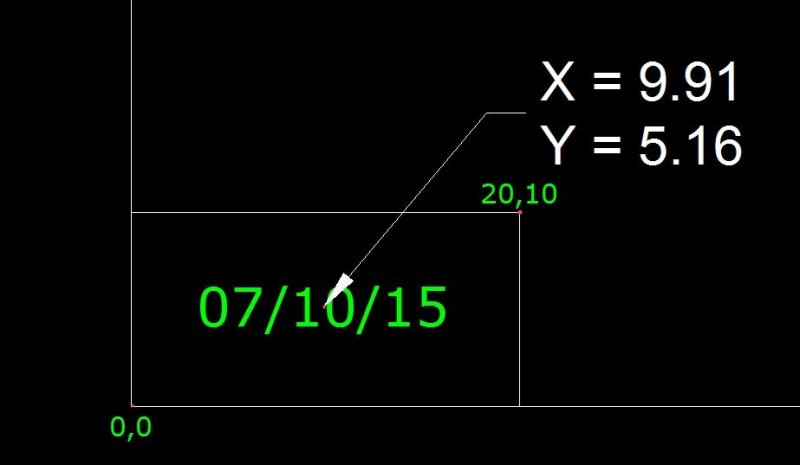# Center Text in Box - From ENTSEL to SSGET?

## Recommended PostsI found this code on the web somewhere and it works great for a single line of text. I would like to be able to select multiple lines of text and have it do the same.

To center several lines of text in an existing rectangle.

I am hoping someone already has code to do this rather than hoping someone

would code it for me. I am clueless on how to do this..Many times what I encounter is multiple lines of individual text items.

So it may be TEXT or it may be MTEXT.

Here is the existing code which works great for a single line of text:

```; PLACE TEXT AT THE CENTER OF A BOX OR A CIRCLE,
; IRRESPECTIVE OF TEXT'S INS.PT
; By G. Ramesh, NPCC, Abu Dhabi.

(defun c:CTT(/ e el p1 p2 mid tb sp ll ur mid1 dis)

(Princ "\nCenter Text in Box. ")

(setvar "cmdecho" 0)

(setq e (car(entsel "\nPick the text: "))
el (entget e))

(initget "Window Circle")

(setq opt (getkword "\nTo be enclosed within a Window/Circle <W>: "))

(if (or (not opt) (= opt "WINDOW"))

(setq p1 (getpoint "\nFirst corner of the enclosing box: ")

p2 (getcorner p1 "\nOther corner: ")

mid (polar p1 (angle p1 p2) (/ (distance p1 p2) 2))
)

(setq mid (cdr(assoc 10 (entget(car(entsel "\nSelect a CIRCLE: "))))))
)

(setq tb (textbox el) sp (cdr(assoc 10 el))

ll (mapcar '+ (car tb) sp)

ur (mapcar '+ ll (mapcar '- (cadr tb) (car tb)))

mid1 (polar ll (angle ll ur) (/ (distance ll ur) 2.0))

dis (mapcar '- mid mid1)
)

(command ".move" e "" dis "")

(prin1)
)
```

Apologies for the formatting being all wonky, it was like that...

Thanks much!

rev

##### Share on other sitesHI

thank you

yathishkumar

CTR2RECT.LSP

##### Share on other sitesThanks for the reply but, that code does the same thing as mine...

(A single line of text)

I want to be able to select multiple lines of text (ie:SSGET)

The code needs to determine the "center point" based off of

how many items were selected and their respective heights.

If it needs to change the justification that would be fine.

##### Share on other sitesI found this code on the Autodesk Forums...

It "somewhat works."

With this code, the text has to lie somewhere inside the rectangle already.

It centers the text but multiple lines are ALL placed in the center on top of each other.

Doesn't work with MTEXT though.

```(defun c:CenterText (/ ss i elast obj bent ipt)
(defun getmid (ent)
(setq lst (mapcar 'cdr (vl-remove-if-not '(lambda (x) (= 10 (car x))) (entget ent))))
(if (= (length lst) 4)
(mapcar '(lambda (a b) (/ (+ a b) 2.)) (car lst) (caddr lst))
)
)

(command "_undo" "begin")
(prompt "\Selext text within the table.")
(setq ss (ssget "_:L" '((0 . "TEXT"))))
(setq i -1)
(while (setq ename (ssname ss (setq i (1+ i))))
(setq obj (vlax-ename->vla-object ename))
(setq elast (entlast))
(setq ipt (vlax-get obj 'insertionpoint))
(if (vl-catch-all-error-p
(vl-catch-all-apply
'(lambda ()
(vl-cmdf "_-BOUNDARY" (trans ipt 0 1) "")
(while (> (getvar "CMDACTIVE") 0) (command ""))
)
)
)
;;  got a boundry
(progn
(if (and (not (eq elast (setq bent (entlast))))
(setq midpt (getmid bent))
)
(progn
(vla-put-Alignment obj acAlignmentMiddleCenter)
(vla-put-TextAlignmentPoint Obj (vlax-3D-point MidPT))
)
(and (eq bent (entlast)) (entdel bent))
)
)
)
)
(command "_undo" "end")

(princ)
)```

##### Share on other sitesSheesh!

Found a modified version of the above code.

This works perfectly IF the text is MTEXT.

I had to add the ERASE command line since it drew in

a bounding box and then left it there.

All you Master-Coder-Dudes probably laugh at my duct tape fix but it works..

It still places individual TEXT items all on top of each other in the center. :-\

So close...

```(defun c:ct2 (/ *error* vl ov i ss ent obj pt prop eLst poly)

(defun *error* (msg)
(and ov (mapcar 'setvar vl ov))
(or (wcmatch (strcase msg) "*BREAK,*CANCEL*,*EXIT*")
(princ (strcat "\n** Error: " msg " **")))
(princ))

(setq vl '("CMDECHO" "OSMODE") ov (mapcar 'getvar vl))
(mapcar 'setvar vl '(0 0))

(if (setq i -1 ss (ssget "_:L" '((0 . "MTEXT,TEXT"))))
(while (setq ent (ssname ss (setq i (1+ i))))
(setq obj (vlax-ename->vla-object ent))

(if (eq "AcDbText" (vla-get-ObjectName obj))
(if (eq AcAlignmentLeft (vla-get-Alignment obj))
(progn
(setq tmp (vla-get-InsertionPoint obj))
(vla-put-Alignment obj acAlignmentMiddleCenter)
(vla-put-TextAlignmentPoint obj tmp))
(vla-put-Alignment obj acAlignmentMiddleCenter))
(vla-put-AttachmentPoint obj acAttachmentPointMiddleCenter))

(setq pt (vlax-get obj
(setq prop
(if (eq "TEXT" (cdr (assoc 0 (entget ent))))
'TextAlignmentPoint 'InsertionPoint))))

(setq eLst (entlast))
(vl-cmdf "_.-boundary" pt "")

(if (not (eq eLst (setq poly (entlast))))
(progn
(vla-getBoundingBox (vlax-ename->vla-object poly) 'MiP 'MaP)

(setq pLst (mapcar 'vlax-safearray->list (list mIP maP)))

(vlax-put-property obj prop
(vlax-3D-point
(polar (car pLst) (apply 'angle pLst) (/ (apply 'distance pLst) 2.))))

(entdel poly))

(mapcar 'setvar vl ov)

(Command "Erase" "L" "")

(princ))```

##### Share on other sitesIf you take multiple lines of text the way you see them is not necessarily the order a ssget will return you need to sort the lines of text say based on the Y value of the text insertion lower left. Once you have this new order list it would be easy to place inside a box. Stuff like line spacing still to be worked out.

Anyone sort this ?

((y1 text1)(y2 text2)(y3 Text3)) correct is ((y2 text2)(y3 text3)(y1 text1))

##### Share on other sitesTry the following program:

```[color=GREEN];; Center Text in Polyline  -  Lee Mac[/color]
([color=BLUE]defun[/color] c:txtrec ( [color=BLUE]/[/color] f i l m n p r s x )
([color=BLUE]if[/color]
([color=BLUE]and[/color]
([color=BLUE]setq[/color] s (LM:ssget [color=MAROON]"\nSelect text or mtext: "[/color] '([color=MAROON]"_:L"[/color] ((0 . [color=MAROON]"TEXT,MTEXT"[/color])))))
([color=BLUE]setq[/color] p (LM:ssget [color=MAROON]"\nSelect polyline: "[/color]  '([color=MAROON]"_+.:E:S"[/color] ((0 . [color=MAROON]"LWPOLYLINE"[/color])))))
)
([color=BLUE]progn[/color]
([color=BLUE]setq[/color] r ([color=BLUE]cdr[/color] ([color=BLUE]assoc[/color] 50 ([color=BLUE]entget[/color] ([color=BLUE]ssname[/color] s 0))))
m ([color=BLUE]list[/color] ([color=BLUE]list[/color] ([color=BLUE]cos[/color] r) ([color=BLUE]sin[/color] r) 0) ([color=BLUE]list[/color] ([color=BLUE]-[/color] ([color=BLUE]sin[/color] r)) ([color=BLUE]cos[/color] r) 0) '(0 0 1))
n ([color=BLUE]list[/color] ([color=BLUE]list[/color] ([color=BLUE]cos[/color] r) ([color=BLUE]-[/color] ([color=BLUE]sin[/color] r)) 0) ([color=BLUE]list[/color] ([color=BLUE]sin[/color] r) ([color=BLUE]cos[/color] r) 0) '(0 0 1))
)
([color=BLUE]repeat[/color] ([color=BLUE]setq[/color] i ([color=BLUE]sslength[/color] s))
([color=BLUE]setq[/color] l
([color=BLUE]append[/color] l
([color=BLUE]mapcar[/color] '([color=BLUE]lambda[/color] ( x ) (mxv m x))
(text-box ([color=BLUE]entget[/color] ([color=BLUE]ssname[/color] s ([color=BLUE]setq[/color] i ([color=BLUE]1-[/color] i)))))
)
)
)
)
([color=BLUE]vl-cmdf[/color] [color=MAROON]"_.move"[/color] s [color=MAROON]""[/color] [color=MAROON]"_non"[/color]
(mxv n
(
([color=BLUE]setq[/color] f
([color=BLUE]lambda[/color] ( l )
([color=BLUE]apply[/color] '[color=BLUE]mapcar[/color]
([color=BLUE]cons[/color] '([color=BLUE]lambda[/color] ( a b ) ([color=BLUE]/[/color] ([color=BLUE]+[/color] a b) 2.0))
([color=BLUE]mapcar[/color] '([color=BLUE]lambda[/color] ( f ) ([color=BLUE]apply[/color] '[color=BLUE]mapcar[/color] ([color=BLUE]cons[/color] f l))) '([color=BLUE]min[/color] [color=BLUE]max[/color]))
)
)
)
)
l
)
)
[color=MAROON]"_non"[/color]
(f
([color=BLUE]mapcar[/color] '[color=BLUE]cdr[/color]
([color=BLUE]vl-remove-if-not[/color] '([color=BLUE]lambda[/color] ( x ) ([color=BLUE]=[/color] 10 ([color=BLUE]car[/color] x)))
([color=BLUE]entget[/color] ([color=BLUE]ssname[/color] p 0))
)
)
)
)
)
)
([color=BLUE]princ[/color])
)

[color=GREEN];; The following function is based on code by gile[/color]

([color=BLUE]defun[/color] text-box ( enx [color=BLUE]/[/color] bpt hgt jus lst ocs org rot wid )
([color=BLUE]cond[/color]
(   ([color=BLUE]=[/color] [color=MAROON]"TEXT"[/color] ([color=BLUE]cdr[/color] ([color=BLUE]assoc[/color] 00 enx)))
([color=BLUE]setq[/color] bpt ([color=BLUE]cdr[/color] ([color=BLUE]assoc[/color] 10 enx))
rot ([color=BLUE]cdr[/color] ([color=BLUE]assoc[/color] 50 enx))
lst ([color=BLUE]textbox[/color] enx)
)
)
(   ([color=BLUE]=[/color] [color=MAROON]"MTEXT"[/color] ([color=BLUE]cdr[/color] ([color=BLUE]assoc[/color] 00 enx)))
([color=BLUE]setq[/color] ocs  ([color=BLUE]cdr[/color] ([color=BLUE]assoc[/color] 210 enx))
bpt  ([color=BLUE]trans[/color] ([color=BLUE]cdr[/color] ([color=BLUE]assoc[/color] 10 enx)) 0 ocs)
rot  ([color=BLUE]angle[/color] '(0.0 0.0) ([color=BLUE]trans[/color] ([color=BLUE]cdr[/color] ([color=BLUE]assoc[/color] 11 enx)) 0 ocs))
wid  ([color=BLUE]cdr[/color] ([color=BLUE]assoc[/color] 42 enx))
hgt  ([color=BLUE]cdr[/color] ([color=BLUE]assoc[/color] 43 enx))
jus  ([color=BLUE]cdr[/color] ([color=BLUE]assoc[/color] 71 enx))
org  ([color=BLUE]list[/color] ([color=BLUE]cond[/color] (([color=BLUE]member[/color] jus '(2 5 ) ([color=BLUE]/[/color] wid -2.0)) (([color=BLUE]member[/color] jus '(3 6 9)) ([color=BLUE]-[/color] wid))      (0.0))
([color=BLUE]cond[/color] (([color=BLUE]member[/color] jus '(1 2 3)) ([color=BLUE]-[/color] hgt))      (([color=BLUE]member[/color] jus '(4 5 6)) ([color=BLUE]/[/color] hgt -2.0)) (0.0))
)
lst  ([color=BLUE]list[/color] org ([color=BLUE]mapcar[/color] '[color=BLUE]+[/color] org ([color=BLUE]list[/color] wid 0)) ([color=BLUE]mapcar[/color] '[color=BLUE]+[/color] org ([color=BLUE]list[/color] wid hgt)) ([color=BLUE]mapcar[/color] '[color=BLUE]+[/color] org ([color=BLUE]list[/color] 0 hgt)))
)
)
)
([color=BLUE]if[/color] lst
(   ([color=BLUE]lambda[/color] ( m ) ([color=BLUE]mapcar[/color] '([color=BLUE]lambda[/color] ( p ) ([color=BLUE]mapcar[/color] '[color=BLUE]+[/color] (mxv m p) bpt)) lst))
([color=BLUE]list[/color]
([color=BLUE]list[/color] ([color=BLUE]cos[/color] rot) ([color=BLUE]sin[/color] ([color=BLUE]-[/color] rot)) 0.0)
([color=BLUE]list[/color] ([color=BLUE]sin[/color] rot) ([color=BLUE]cos[/color] rot)     0.0)
'(0.0 0.0 1.0)
)
)
)
)

[color=GREEN];; ssget  -  Lee Mac[/color]
[color=GREEN];; A wrapper for the ssget function to permit the use of a custom selection prompt[/color]
[color=GREEN];; msg - [str] selection prompt[/color]
[color=GREEN];; arg - [lst] list of ssget arguments[/color]

([color=BLUE]defun[/color] LM:ssget ( msg arg [color=BLUE]/[/color] sel )
([color=BLUE]princ[/color] msg)
([color=BLUE]setvar[/color] 'nomutt 1)
([color=BLUE]setq[/color] sel ([color=BLUE]vl-catch-all-apply[/color] '[color=BLUE]ssget[/color] arg))
([color=BLUE]setvar[/color] 'nomutt 0)
([color=BLUE]if[/color] ([color=BLUE]not[/color] ([color=BLUE]vl-catch-all-error-p[/color] sel)) sel)
)

[color=GREEN];; Matrix x Vector  -  Vladimir Nesterovsky[/color]
[color=GREEN];; Args: m - nxn matrix, v - vector in R^n[/color]

([color=BLUE]defun[/color] mxv ( m v )
([color=BLUE]mapcar[/color] '([color=BLUE]lambda[/color] ( r ) ([color=BLUE]apply[/color] '[color=BLUE]+[/color] ([color=BLUE]mapcar[/color] '[color=BLUE]*[/color] r v))) m)
)

([color=BLUE]princ[/color])
```

The above should work with both Text & MText at any rotation angle & with any justification.

If the text is to be aligned, use my Align Text program before using the above (and use the JUSTIFYTEXT command before that if the text has varying justification settings).

Assumptions & Notes:

• Assumes all text in the selection has the same rotation.
• Assumes WCS (not tested/designed for all UCS/View settings)

##### Share on other sitesThanks much Lee!!

I will check this out as soon as I get back to the office...

##### Share on other sitesYou're welcome - I hope all goes well##### Share on other sitesHi lee,sir

thank you very much.

i'ts working super.

thank you

yathishkumar

##### Share on other sitesExcellent, thank you for the feedback yathishkumar.##### Share on other sitesIs it possible to change this to centre the text/mtext between two selected points?

Sometimes it's desirable to centre the *text to part of a block or a non rectangular polygon or even simply between two entities.

I did try to work out where the second part of the move command was, but I'm baffled

Thanking someone in anticipation

- Harry

##### Share on other sitesIs it possible to change this to centre the text/mtext between two selected points?

No time to test it, but try changing this:

`            (setq p (LM:ssget "\nSelect polyline: "  '("_+.:E:S" ((0 . "LWPOLYLINE")))))`

to:

```            (setq p (getpoint "\nSpecify 1st point: "))
(setq q (getpoint "\nSpecify 2nd point: " p))```

And this:

```                    (mapcar 'cdr
(vl-remove-if-not '(lambda ( x ) (= 10 (car x)))
(entget (ssname p 0))
)
)```

to:

`                    (list p q)`

##### Share on other sitesLee, you're a genius

Thanks v v much, the lisp covers all instances of needing to put *text to the MC of entities

##### Share on other sitesThank you Happy Hobbit, I'm glad it helps!

##### Share on other sitesThat's peculiar, I'm sure that it worked great yesterday. After using the modified lisp a few times I notice a slight error.

Please Lee, if you ever find a spare few minutes....##### Share on other sitesAre you reading the coordinates of the text alignment point or the center of the bounding box of the text object?

Note that the program is positioning the text based on the center of the bounding box, not the text alignment point.

##### Share on other sitesThe leader is on the alignment point..

I was just thinking that the lisp calculates the centre of the text from the overall size of the text entity, in which of course the insertion point may not be the same as the centre of the text depending what characters are within the string.

##### Share on other sitesLee...

I just realized that I had never gotten back after trying this routine out...Like all your stuff, it works great!

For this and all that you have helped me with over the years...

THANK YOU!!

##### Share on other sitesNo problem at all ILoveMadoka - you're most welcome!

The program was fun to write and so I'm glad it proved useful as well!

## Join the conversation

You can post now and register later. If you have an account, sign in now to post with your account.
Note: Your post will require moderator approval before it will be visible.×   Pasted as rich text.   Paste as plain text instead

Only 75 emoji are allowed.